# Estimation of Rotor Position in Brushless Direct Current Motor by Memory Attenuated Extended Kalman Filter

Estimation of Rotor Position in Brushless Direct Current Motor by Memory Attenuated Extended Kalman Filter
School of Mechanical and Electrical Engineering, Zaozhuang University, Zaozhuang 277160, China

Corresponding Author Email:
yins8866@yeah.net
Page:
35-42
|
DOI:
https://doi.org/10.18280/ejee.210106
29 December 2018
|
Accepted:
5 February 2019
|
Published:
15 April 2019
| Citation

OPEN ACCESS

Abstract:

The Kalman filter suffers from instability and divergence in the estimation of rotor position in brushless direct current (DC) motor, owing to the time-variation of motor parameters and the distortion of the mathematical model. To solve the problem, this paper presents a new rotor position estimation method using memory attenuated extended Kalman filter (MAEKF). The filter divergence caused by model error and calculation error was suppressed by adjusting the gain of the MAEKF. A motor control system was designed based on the MAEKF, by determining the rotor position angle and the order of conduction of motor windings. The simulation and experiments show that the motor controlled by this method provided accurate commutation signals under no load, load and sudden change of load torque; the system ran stably without divergence; the control system achieved strong robustness.

Keywords:

brushless direct current (DC) motor, Kalman filter, sensorless controller, commutation

1. Introduction

Brushless DC motor is finding wider and wider application in the industry thanks to its high efficiency and ease of control. Its rotor adopts a permanent magnet steel that functions as generating a magnetic field in the air gap of the motor; its stator is an armature winding that generates a response magnetic field when there is a power. In order to generate a torque drive rotor in a single direction, the magnetic fields set up by permanent magnet steel of rotor and armature response of stator must be perpendicular to each other. In this case, the control system is required to identify the position of the current rotor. Today, most of brushless DC motors use the Hall position sensors for the rotor, but things go wrong, e.g. there are high cost, large footprint, and poor reliability. So, scholars all over the world have been hammering at the position sensorless controller .

Sensorless controller is a hotspot to study the brushless DC motor [2-5]. For example, Kiree designs a speed PID control system based on particle swarm optimization for brushless DC motor, which detects the counter electromotive force of the motor to gain the position information about rotor, and shows how the speed and the torque of the motor respond to in the cases of no-load and full-load. Yin proposes a rotor position detection method based on the line voltage, which calculates the back electromotive force of the motor by obtaining the line voltage waveform to determine its commutation point.

As an optimal state, Kalman filter has been widely applied to the estimation of rotor position in brushless DC motors [7-11]. For example, Terzic adopts the extended Kalman filter to estimate the motor rotor position by measuring the line voltage and phase current. Zhang devises a sensorless control program for brushless DC motor, i.e. a dual extended Kalman filter, by which the motor state and parameters are filtered in parallel to eliminate filter divergence caused by inaccurate motor parameters. Shi develops the sensorless BLDCM speed controller based on EKF phase gain calibration, and emendates the phase lag and amplitude distortion of the counter electromotive force against different speeds. The motor rotor relevant position information will be available from the back EMF normalization as observed using an extended Kalman filter (EKF) estimator.

There are gaps of the brushless DC motor, for example, it is not easy to measure relevant parameters, the motor runs unstably, and there are errors in the motor model, when using a Kalman filter, it is prone to filter divergence, poor filter precision, and slow filtering. The MAEKF, if available, can ensure the filter convergence and stability by slipping old measured values from its memory but using new values [12-14].

Based on the analysis of the brushless DC motor model, the motor rotor position angle and speed are estimated by the MAEKF after the motor phase current is measured. Then the motor commutation will be realized based on the mapping relation between rotor position angle and motor commutation strategy. The method has a strong robustness since it has a low requirement on the accuracy of the system model and parameters.

2. Control Strategy for Brushless DC Motor

Brushless DC motor is a variant with permanent magnet as rotor and AC coil as stator. Unlike ordinary DC motor which, whenever the permanent magnet and coil of DC motor generate the torque in a different direction from stable torque as required, uses the carbon brush of the mechanical structure for commutation to make the electromagnetic torque generated by the coil continue to provide a stable electromagnetic torque, the brushless DC motor is essentially a system that consists of motor body, rotor position detector unit which detects rotor signal, and current inverter unit which performs electronic commutation depending on the rotor position signals to ensure that the AC magnetic fields generated by the stator magnetic field and coil excitation are always perpendicular to each other when the brushless DC motor runs normally.

Subject to the special structure of the brushless DC motor, there are three control methods for the brushless DC motor, i.e. three-phase half-control circuit, three-phase star type full control circuit, and three-phase triangular full control circuit, of which, the full control circuit can be divided into two-way and three-way conduction modes. In the three-phase half-control circuit, it takes only one-third of the total time for each phase to be breakover. There are high motor efficiency and torque ripple coefficient of motor. The two-way conduction mode in three-phase full controller circuit is a more common used control program, that is, only two phases of winding can be breakover every time it is energized. In practice, two-way conduction mode is most used. The three-phase star type full control circuit under two-way control mode is shown in Figure (1). The motor controller unit consists of 6 MOSFET power tubes.

## figure_1.png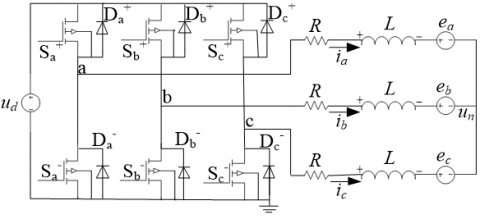Figure 1. Equivalent model of the star connection BLDCM

Take the commutation from phases ab to ac as an example, the working process of the MOSFET power tube in the two-way control mode is explained. When the phase ab is turned on, transistors Sa+ and Sb-MOSs are active, and the remaining transistors MOS are inactive. When the commutation signal is detected, the transistor MOS is activated by Sa+ and Sc- instead of Sa+ and Sb- which are active originally. In this way, the motor fulfils a commutation.

## figure_2.png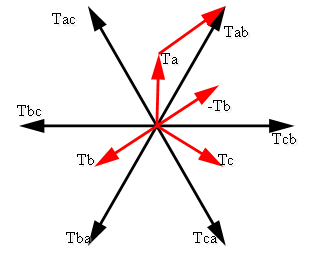Figure 2. Schematic diagram of ab-phase resultant torque

When the brushless DC motor is commutating, if the electromagnetic torque generated by the current direction, as shown in the figure, is considered to be a positive value, otherwise, it is a negative value . Then, when the phase ab is turned on, Sa+Sb- in the MOS transistor is activated. The current flows in the reference direction, as shown in the figure, from the phase a winding, and in the opposite direction from the phase b winding. At this time, the electromagnetic torques generated by a- and b-phase windings are positive and negative values, respectively. Owing to the internal rotor structure of the motor, the generated torque directions are shown in Figure 2. The torque is $\sqrt{3}T_a$. When the motor turns by an electrical angle of 60 degrees, the MOS transistor is switched on by Sc-Sb+. Among them, the phase BC winding is energized; the B-phase winding current follows the reference direction in the figure, while the C-phase winding has a current opposite to the reference direction. The resultant torque, as shown in Figure 2, is identical to Sa+Sc- in size, and follows the direction deviated 60 degrees from that when Sc-Sb+is active.

In the two-way commutation mode, two-phase windings are turned on at each commutation time, and the brushless DC motor is commutated once every 1/6 cycle. That is to say, the motor is commutated once every 60 degrees electrical angle; each MOS transistor is turned on by 120 degrees, and each phase winding is activated by 240 degrees.

The rotor magnet steel rotates to drive the magnetic field created by it also to spin. At this time, the rotating magnetic field in turn cuts the winding coil. According to the law of electromagnetic induction, the alternating magnetic field will generate the induced electromotive force in the winding. This is the origin of the back EMF of brushless DC motor. However, the back EMF, if available, will interfere with the balance of back EMF in the motor. Thus there is a mapping relation between the alternating magnetic field, the phase current, and the back EMF in the motor, which can provide detection conditions for the commutation of the brushless DC motor during operation.

3. Model of Brushless DC Motor

3.1 Mathematical model

The magnetic induction intensity generated by the stator magnet of the brushless DC motor at any time can be expressed as:

${{B}_{\delta }}=\frac{\Phi \left( \theta \right)}{\tau \cdot L}$ (1)

where, $Φ(θ)$ is the magnetic flux for each pole winding at the rotor position angle $θ$; τ is the pole distance; L is the active length of the motor core; $τ⋅L$ is the active area of each winding.

The permanent flux linkage of a winding turn (the flux linked in the winding coil during an electrical angular period) can be expressed as the flux linked in the winding coil at each electrical angle, and therefore, in the case when the rotor position angle is $α'$, the permanent flux linkage of a turn of winding is expressed as:

${{\phi }_{pn}}\left( \alpha \right)=\int_{-\frac{\pi }{2}+\alpha }^{\frac{\pi }{2}+\alpha }{{{B}_{\delta }}\left( \theta \right)\cdot sd\theta }$ (2)

where,  $B_δ(θ)$ is the winding magnetic induction intensity when the rotor position angle is θ;  $s=τ⋅L$ is the active area of each winding.

The permanent flux linkage of the a-phase winding can be expressed as:

${{\Phi }_{a}}={{\phi }_{pn}}\left( {{\alpha }'} \right)\cdot N$ (3)

where, N is the number of a-phase winding turns.

It is known from the law of electromagnetic induction that the relative motion of the rotor magnetic field to the stator winding generates an induced electromotive force equal to the gradient of magnetic flux passing through the stator winding, and the winding induction potential can be expressed as:

${{e}_{{{\Phi }_{a}}}}=\frac{d{{\Phi }_{a}}}{dt}$ (4)

Now the interference of uneven air gap and edge diffusion in the air gap magnetic induction intensity can be neglected, the air gap magnetic induction intensity of the brushless DC motor can be considered to be trapezoidal distribution along the inner diameter surface of the stator.

Equations (1)~(3) are substituted into Equation (4), the back EMF of phase a winding can be expressed as:

$e_a=\frac{d}{dt}[N\int_{-\frac{π}{2}+α'}^{\frac{π}{2}+α'}B_δ(θ)⋅sdθ]=N⋅S⋅ω⋅[B_δ(\frac{π}{2}+θ)-Bδ(-\frac{π}{2}+θ)]=2⋅N⋅S⋅ω⋅B_δ(\frac{π}{2}+θ)$ (5)

where, $ω$ is the electrical angular velocity of motor.

It is found from (5) that there is a difference of 90 degrees between the back EMF of a phase winding and the magnetic induction intensity, which show a trapezoidal distribution around the rotor position angle.

The winding phase voltage of the brushless DC motor is made up of the inter-turn mutual induced EMF from self-induced EMF evoked by resistance drop, alternating current, and the induced EMF evoked by variation in the magnetic flux of winding. Take the a-phase winding as an example, the phase voltage of the a-phase winding can be expressed as:

${{u}_{a}}=R{{i}_{a}}+\left( L-M \right)\frac{d{{i}_{a}}}{dt}+{{e}_{a}}+{{u}_{n}}$ (6)

where, R is each phase winding resistance; L is the a-phase winding self-inductance; M is the mutual induction between a-phase winding and b-phase and c-phase winding; ia is the a-phase winding current; uN is the neutral point voltage. The phase voltages of b-phase and c-phase windings are similar to that of a-phase, and the three can all be expressed as:

$\begin{bmatrix} u_a \\ u_b \\ u_c \end{bmatrix}=\begin{bmatrix} R & 0 & 0 \\ 0 & R & 0 \\ 0 & 0 & R \end{bmatrix} \begin{bmatrix} i_a \\ i_b \\ i_c \end{bmatrix}+\begin{bmatrix} L_S & 0 & 0 \\ 0 & L_S & 0 \\ 0 & 0 & L_S \end{bmatrix} \frac{d}{dt} \begin{bmatrix} i_a \\ i_b \\ i_c \end{bmatrix}+\begin{bmatrix} e_a \\ e_b \\ e_c \end{bmatrix}+\begin{bmatrix} u_n \\ u_n \\ u_n \end{bmatrix}$  (7)

where, ua, ub, uc are the phase voltages of three phase windings, respectively; ia, ib, ic are their respective currents; ea, eb, ec are their respective back EMFs; R is the resistance of each phase winding; Ls is the difference between self-inductance and mutual inductance, $L_s=L-M$.

The line voltage equation can be expressed as:

$\begin{bmatrix} u_{ab} \\ u_{bc} \\ u_{ca} \end{bmatrix}=\begin{bmatrix} R & -R & 0 \\ 0 & R & -R \\ -R & 0 & R \end{bmatrix} \begin{bmatrix} i_a \\ i_b \\ i_c \end{bmatrix}+\begin{bmatrix} L_S & 0 & 0 \\ 0 & L_S & 0 \\ 0 & 0 & L_S \end{bmatrix} \frac{d}{dt} \begin{bmatrix} i_a \\ i_b \\ i_c \end{bmatrix}+\begin{bmatrix} e_{a_b} \\ e_{b_c} \\ e_{c_a} \end{bmatrix}$ (8)

where, uab, ubc, uca are the line voltages of three phase windings, respectively.

When the mechanical and stray losses of the motor are neglected, the BLDCM’s electromagnetic power is converted into the kinetic energy of the rotor for work. The torque of the brushless DC motor can be expressed as:

$P={{T}_{e}}\omega$ (9)

The electromagnetic power of the motor is equal to the sum of the products of the back EMFs of the three phase windings and the phase currents, namely:

$P={{e}_{a}}{{i}_{a}}+{{e}_{b}}{{i}_{b}}+{{e}_{c}}{{i}_{c}}$  (10)

After substituting it into the above equations, then:

${{T}_{e}}={\left( {{e}_{a}}{{i}_{a}}+{{e}_{b}}{{i}_{b}}+{{e}_{c}}{{i}_{c}} \right)}/{\omega }\;$ (11)

where, Te  is the electromagnetic torque; ω is the mechanical angular velocity of the motor;

After substitute Form 5 into Form 11, the electromagnetic torque can be expressed as:

${{T}_{e}}={{\lambda }_{m}}\left( {{B}_{\delta a}}{{i}_{a}}+{{B}_{\delta b}}{{i}_{b}}+{{B}_{\delta c}}{{i}_{c}} \right)$  (12)

where, $λ_m=2NS$ ,  $B_δ$ is the waveform function for the back EMF.

The motor motion equation is given as:

${{T}_{e}}-{{T}_{L}}=J\frac{d\omega }{dt}+{{B}_{v}}\omega$  (13)

where, TL is the load torque; J is the rotor moment of inertia; Bv is the viscous friction coefficient.

The relationship between the rotor position angle and the speed of the motor can be expressed as:

$\frac{d\theta }{dt}=\frac{p}{2}\omega$  (14)

3.2 MAEKF state model of BLDCM

The state equation for the MAEKF model of BLDCM can be available by algebraic transformation using Equations 7, 13, and 14. Here, the three-phase current, motor speed and rotor position angle are chosen as state variables, and the MAEKF state model of BLDCM is available as follows:

${{x}_{k+1}}={{\Phi }_{k}}{{x}_{k}}+{{R}_{k}}{{u}_{k}}$  (15)

where, $x_k=[i_a, i_b, i_c, ω, θ]^T$

$Φ_k=\begin{bmatrix}-\frac{R}{L_s} & 0 & 0 & -\frac{λ_mB_{δa}}{L_s} & 0 \\ 0 & \frac{R}{L_s} & 0 & 0 & 0 \\ 0 & 0 & -\frac{R}{L_s} & 0 & 0 \\ \frac{λ_mB_{δa}}{L_s} & \frac{λ_mB_{δb}}{L_s} & \frac{λ_mB_{δc}}{L_s} & -\frac{B_V}{J} & 0 \\ 0 & 0 & 0 & \frac{p}{2} & 0 \end{bmatrix}$

$R_k=\begin{bmatrix} \frac{1}{L_s} & 0 & 0 & 0 & 0 \\ 0 & \frac{1}{L_s} & 0 & 0 & 0 \\ 0 & 0 & \frac{1}{L_s} & 0 & 0 \\ 0 & 0 & 0 & -\frac{1}{J} & 0 \end{bmatrix}$

$u_k=[u_a, u_b, u_c, T_L]^T$

4. MAEKF Senseless Control System

4.1 MAEKF principle

The MAEKF greatly differ from traditional Kalman filter in that the attenuation factor α is introduced. Thus, the MAEKF is less sensitive to the previous measurement value, and puts its focus on the most recent measurements to allow the filter more concern the existing values. Although added attenuation factor leads to the loss of theoretical optimality in Kalman filter, its stability and convergence are better.

Like other motors, the brushless DC motor also supports some parameter variables such as winding resistance, inductance, and winding inter-turn mutual induction, flux linkage which are subjected to change with the operation of motor, eventually leading to the distortion of mathematical model of brushless DC motor since it also build on the eddy current loss, hysteresis loss, slot effect.

During the operation of the motor, the measured back-EMF changes abruptly due to the conduction of the freewheeling diode at the time of motor commutation, resulting in a false judgement. Since the unmodeled, measured and sudden noises contained in measured back-EMF when motor operates may also cause zero crossing point of the back-EMF, futher the misjudgment may occur. When designing the extended Kalman filter, various noises in the back-EMF are considered as the Gaussian white noises to be processed.

Therefore, the brushless DC motor filter is theoretically subprime, less sensitive to the model and more robust.

For a discrete linear system, there are the following state equations:

${{x}_{k+1}}={{\Phi }_{k}}{{x}_{k}}+{{R}_{k}}{{u}_{k}}+{{G}_{k}}{{w}_{k}}$  (16)

${{y}_{k}}={{H}_{k}}{{x}_{k}}+{{Q}_{k}}{{v}_{k}}$ (17)

where, w(k) is the random input disturbance of system; v(k) is the measured noise of system; w(k), v(k) are all white noises with zero mean and irrelevant,

${{H}_{k}}=\left[ \begin{matrix} 1 & 0 & 0 & 0 & 0 \\ 0 & 1 & 0 & 0 & 0 \\ 0 & 0 & 1 & 0 & 0 \\ \end{matrix} \right]$ .

The covariance matrixes $G_k$ and $Q_k$ of the system random perturbation and measured noises as given in the Literature  are:

${{G}_{k}}=diag\left[ \begin{matrix} 0.4 & 0.4 & 0.4 & 3.16 & 20 \\ \end{matrix} \right]$

${{Q}_{k}}=diag\left[ \begin{matrix} 0.5 & 0.5 & 0.5 \\ \end{matrix} \right]$

At k, the system estimate equation can be expressed as:

${{\hat{X}}_{k+1|k}}={{\Phi }_{k}}{{\hat{X}}_{k|k-1}}+{{K}_{k}}\left( {{y}_{k}}-{{H}_{k}}{{{\hat{X}}}_{k|k-1}} \right)$  (18)

where, Kk is the gain of MAEKF. It can determine the offset of filter estimate from that value in the real state, that is, the greater the value Kk, the closer the estimate is to the system's measured value, otherwise, the closer it is to the system's calculated value.

The optimal standard for the gain of the Kalman filter is to minimize the variance of the estimate error at k, that is, to obtain the Kalman filter gain by minimizing E(JN). Here JN is:

$J_N=\sum^N_{K=1}$$[(y_k-H_k)\hat{X}_{k|k-1})^TR^{-1}_k(y_k-H_k)\hat{X}_{k|k-1})+\hat{W}^T_kQ^{-1}_k\hat{w}k]$  (19)

Here, we can find the right filter by minimizing $E(\tilde{J}_N)$. $\tilde{J}_N$ is defined as:

$\tilde{J}_N=\sum^N_{K=1}[(y_k-H_k)\hat{X}_{k|k-1})^Tα^{2k}R_k^{-1}(y_k-H_k)\hat{X}_{k|k-1})+\hat{W}^T_kα^{2k+2}Q_k^{-1}\hat{w}k]$  (20)

where, the first α of the cost function minimizes the covariance of the current moment, which makes for the state estimate of the final convergence of the filter to be closer to the new measurement value of the system; the second α of the cost function only facilitates mathematical process, and considered as constant when minimizing the evaluation.

The gain of improved Kalman filter is

${{K}_{k}}={{\alpha }^{2k}}{{P}_{k|k-1}}H_{k}^{T}{{\left( {{H}_{k}}{{P}_{k|k-1}}H_{k}^{T}+{{R}_{k}} \right)}^{-1}}$ (21)

The renewal equation for estimate error covariance is

${{P}_{k-1|k}}={{\Phi }_{k-1}}{{\alpha }^{2k}}{{P}_{k-1|k-2}}\Phi _{k-1}^{T}+{{Q}_{k-1}}$  (22)

The estimation for estimate error covariance is expressed by

${{P}_{k|k-1}}={{\alpha }^{2k}}{{P}_{k-1|k}}-{{K}_{k}}{{H}_{k}}{{P}_{k-1|k}}$ (23)

4.2 Framework of MAEKF control system

The framework of the position sensorless control system for brushless DC motor using MAEKF is shown in Figure 3.

## figure_3.png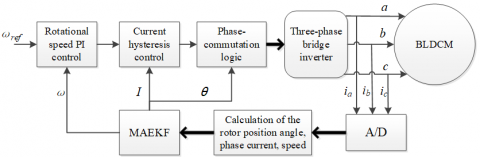Figure 3. Framework of MAEKF controller

The proposed control system mainly consists of five parts: speed PI controller, hysteresis current controller, motor parameter counter, MAEKF filter, and brushless DC motor. It samples the three-phase winding current (ia, ib, ic), and obtains them via AD conversion as the input of the model. There are state variables, i.e. the three-phase winding current, the speed ω and the electrical angle θ. It is possible to determine the commutation time for the motor according to the rotor position relation. The job relation of the said electrical angle with motor winding and power device is shown in Table 1.

Table 1. Job relation of the electrical angle with motor winding and power device

 Chapter 1 Electrical Angle θ Chapter 2 Winding State Chapter 3 Power Devices State Chapter 4 0˚~60˚ Chapter 5 AB phase conduction Chapter 6 Sa+ and Sb- conduction Chapter 7 60˚ Chapter 8 Turn off AB phase and turn on AC phase Chapter 9 Turn off Sb- and turn on Sc- Chapter 10 60˚~120˚ Chapter 11 AC phase conduction Chapter 12 Sa+、Sc- conduction Chapter 13 120˚ Chapter 14 Turn off AC phase and turn on BC phase Chapter 15 Turn off Sa+ and turn on Sb+ Chapter 16 120˚~180˚ Chapter 17 BC phase conduction Chapter 18 Sb+、Sc- conduction Chapter 19 180˚ Chapter 20 Turn off BC phase and turn on BA phase Chapter 21 Turn off Sc- and turn on Sa- Chapter 22 180˚~240˚ Chapter 23 BA phase conduction Chapter 24 Sb+、Sa- conduction Chapter 25 240˚ Chapter 26 Turn off BA phase and turn on CA phase Chapter 27 Turn off Sa- and turn on Sc+ Chapter 28 240˚~300˚ Chapter 29 CA phase conduction Chapter 30 Sc+、Sa- conduction Chapter 31 300˚ Chapter 32 Turn off CA phase and turn on CB phase Chapter 33 Turn off Sa- and turn on Sc+ Chapter 34 300˚~360˚ Chapter 35 CB phase conduction Chapter 36 Sc+、Sb- conduction Chapter 37 360 ˚ Chapter 38 Turn off CB phase and turn on AB phase Chapter 39 Turn off Sc+ and turn on Sa+
4.3 Simulation

The MAEKF-based position sensorless controller model for brushless DC motor is established in Matlab/Simulink. It adopts the PI controller to control the speed and the hysteresis controller to control the current. In the simulation, the prototype parameters include the rated DC side voltage ud=24V; rated torque TN =0.18 N·m; rated speed nN =2000 r/min; phase resistance R =1.2 Ω; inductance L =1.2 mH, attenuation factor α=1.036.The motor run at no load and is applied suddenly with the torque load of 0.1 N·m at 0.4s. The simulation results are shown in Figures 4-6.

## figure_4.pngFigure 4. Motor speed, torque and rotor position angle waveforms when load torque changes abruptly

## figure_5.png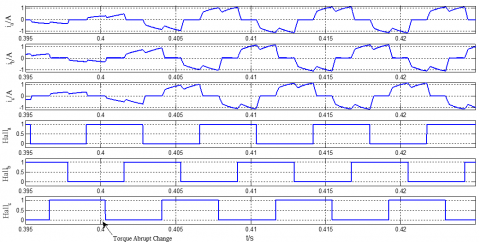Figure 5. Phase current and Hall signal waveforms when load torque changes abruptly

## figure_6.pngFigure 6. Speed, torque and rotor position angle waveforms when running under loads

The measurement waveform of the motor torque and rotor position signal at the time of sudden change of load torque is shown in Figure 4. As seen from the figure, the motor speed sharply drops as the load torque suddenly changes, but the speed basically reaches the preset value at 0.2s. This dynamic response character is true under the general working conditions. The waveforms of the three-phase currents ia, ib, ic and Hall signals Halla, Hallb, Hallc at the time of sudden change in load torque are shown in Figure 5. It is obvious that the change in the load torque leads the phase current to increasing, and extending its cycle. The electromagnetic torque generated with the increase of the current builds up to realize the balanced load torque. The results from the simulation test show that the motor can accurately capture the rotor position signal when the load torque changes abruptly. It does not stall and there is no phase-deficient operation. To sum up, this method can still operate stably even if the motor load torque changes suddenly.

Under 0.1N·m load torque, the relationship among speed, torque and rotor position angle of the motor when it runs for a long time is shown in Figure 6. When the load torque is constant, the motor speed reaches the preset value, and the steady-state error is zero; the electromagnetic torque is a specified value, and the output torque ripple is the commutation torque pulsation caused by the discontinuous change in phase current when commutating; the measured motor rotor position angle continuously changes. The simulation results reveal that the filter divergence will not be subject to the model accuracy even when the system runs for a long time under loads.

The motor never stalls when operating for a long time under loads and the load torque changes. The speed, torque and rotor position angle continuously output. There is a correct mapping relation between the phase current and Hall signal. The MAEKF will be able to estimate the motor rotor position, and correctly give the commutation point for motor in the cases when load torque abruptly changes and is constant. There is also no commutation error. It is thus proved that the method has a strong robustness, and is reliable as it is in simulation test.

5. Test Verification

In order to test whether the above model is feasible, we prepare a test system where there are a TMS320F2812 DSP processor as the main control unit, a three-phase full-bridge drive unit composed of IGBTs and a star-connected brushless DC motor as the controlled objects. The system uses the Hall element ACS758LCB that converts the phase current into voltage signal input into DSP digital-to-analog conversion module by a voltage follower after impedance matching, then the phase current is available by AD sampling. In the test, the parameters of the experimental motor are the same as those of the above simulation.

In the test, the motor is started at no load, and a 0.1 N·m load is suddenly applied at 0.4s after it is free accelerated to be steady state. The waveforms of the measured motor speed, and phase current when the load changes abruptly are shown in Figures 7-8.

## figure_7.pngFigure 7. Measured motor speed waveform

As shown in Figure 7, the speed during the startup of motor is not overshot, and it directly accesses to the steady state. The motor response time is in no time, only 7ms. When the load torque of 0.1 N·m is suddenly applied, the motor speed decreases, but does not change abruptly, and timely restores to the preset value.

## figure_8.pngFigure 8. Phase current waveform when load torque changes abruptly

When the load torque suddenly increases, the phase current period gets longer. The increase of phase current causes the electromagnetic torque to build up to balance the load torque. During the test, there is no sudden change of speed and pull-out torque when the load torque changes suddenly. Test shows that the method can well estimate the rotor position at the time of sudden change of load torque so as to realize an accurate commutation.

## figure_9.png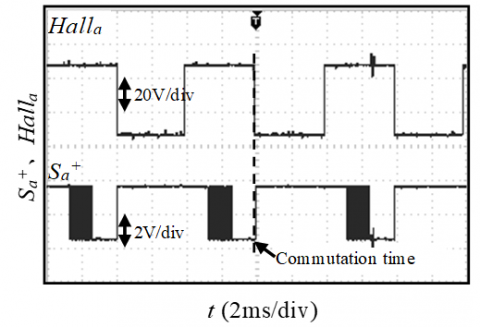Figure 9. Motor Hall signal and power device action waveforms

The action relationship between the motor Hall signal and the power device is shown in Figure 9. From the measured waveforms, we learn that the power device operates slightly behind the Hall signal at a very short hysteresis time, only a few microseconds. Although the sudden change of load torque will lead to a sharp drop of the speed and interfere with the phase current waveform, the system can accurately estimate the rotor position angle without any interference, and give a correct commutation point. Test results bear out the feasibility of the control method.

6. Conclusions

Here we propose a MAEKF-based sensorless controller for brushless DC motor, which enables the estimation on the motor rotor position angle and speed by measuring the motor phase current. After the test, the following conclusions are drawn:

1 The method can well give the rotor position signal in the cases when there is a constant load and the torque changes abruptly. With this method, the motor runs stably and there is no stall and other poor phenomena appeared, and the control system has a strong robustness against motor parameter perturbation and model error, etc. It is therefore proved that the method has high reliability and practicability.

2 The method has a low requirement for motor parameters and model accuracy, but it renders its service at a higher accuracy for parameter measurement. During the commissioning, the attenuation factor can be adjusted according to the system conditions to balance the interference of the model inaccuracy and the parameter measurement error in the system, thus to achieve the system optimality.

References

 Acarnley PP, Watson JF. (2006). Review of position-sensorless operation of brushless permanent-magnet machines. IEEE Transactions on Industrial Electronics  53(2): 352-362. https://doi.org/10.1109/TIE.2006.870868

 Kiree C, Kumpanya D, Tunyasrirut S. (2016). PSO-based optimal PI(D) controller design for brushless DC motor speed control with back EMF detection. Journal of Electrical Engineering & Technology 11(3): 715-723.

 Tong G, Liu Y, Yan Z, Liu Y. (2012). A novel method for estimating the initial rotor position of brushless direct current motor. Procedia Engineering 29: 1133-1136. https://doi.org/10.1016/j.proeng.2012.01.100

 Zhou X, Chen X, Peng C, Zhou YP. (2018). High performance non-salient sensorless BLDC motor control strategy from standstill to high speed. IEEE Transactions on Industrial Informatics 14(10): 4365-4375. https://doi.org/10.1109/TII.2018.2794461

 Jang GH, Park JH, Chang JH. (2002). Position detection and start-up algorithm of a rotor in a sensorless BLDC motor utilising inductance variation. IEE Proceedings - Electric Power Applications 149(2): 137-142. https://doi.org/10.1049/ip-epa:20020022

 Yin S, Ma TH, Lu CQ. (2016). A novel scheme of sensing rotor position of BLDCM based on the line voltage. Small & Special Electrical Machines 44(2): 37-40, 49. https://doi.org/10.3969/j.issn.1004-7018.2016.02.010

 Terzic B, Jadric M. (2002). Design and implementation of the extended Kalman filter for the speed and rotor position estimation of brushless DC motor. IEEE Transactions on Industrial Electronics 48(6): 1065-1073. https://doi.org/10.1109/41.969385

 Zhang Y, Zhao J, Guo Y, Li J. (2010). Improving AECCMA (adaptive error-constrained constant modulus algorithm) for blind equalization to make it suitable in α stable noise. Xibei Gongye Daxue Xuebao/Journal of Northwestern Polytechnical University 28(2): 197-201.

 Shi TN, Zhang Q, Xia CL, Song P, Wan JR. (2008). Estimates of rotor position and velocity of brushless DC motor with UKF algorithm. Journal of Tianjin University 41(3): 338-343. https://doi.org/10.3724/SP.J.1001.2008.01707

 Brunsbach BJ, Henneberger G, Brunsbach BJ. (1990). Field-oriented control of an induction motor without mechanical sensors using a Kalman-Filter. Archiv Für Elektrotechnik 73(5): 325-335.

 Lenine, Reddy R, Kumar V. (2007). Estimation of speed and rotor position of BLDC motor using extended Kalman filter. Iet-uk International Conference on Information & Communication Technology in Electrical Sciences. IET, 2007.

 Hu G, Gao S, Zhong Y, Gao B. (2015). Modified strong tracking unscented Kalman filter for nonlinear state estimation with process model uncertainty. International Journal of Adaptive Control and Signal Processing 29(12): 1561-1577. https://doi.org/10.1002/acs.2572

 Li L, Yu D, Xia Y, Yang HJ. (2017). Stochastic stability of a modified unscented Kalman filter with stochastic nonlinearities and multiple fading measurements. Journal of the Franklin Institute 354(2): 650-667. https://doi.org/10.1016/j.jfranklin.2016.10.028

 Gurung H, Banerjee A. (2016). Self-sensing shape memory alloy wire actuator based on unscented Kalman filter. Sensors & Actuators A Physical 251: 258-265. https://doi.org/10.1016/j.sna.2016.09.037